# Comparison of PCA, NNMF, and ICA over image de-noising

## Introduction

In a previous blog post, , I compared Principal Component Analysis (PCA) / Singular Value Decomposition (SVD) and Non-Negative Matrix Factorization (NNMF) over a collection of noised images of digit handwriting from the MNIST data set, , which is available in Mathematica.

This blog post adds to that comparison the use of Independent Component Analysis (ICA) proclaimed in my previous blog post, .

## Computations

The ICA related additional computations to those in  follow.

First we load the package IndependentComponentAnalysis.m :

``````Import["https://raw.githubusercontent.com/antononcube/\
MathematicaForPrediction/master/IndependentComponentAnalysis.m"]``````

From that package we can use the function `IndependentComponentAnalysis` to find components:

``````{S, A} = IndependentComponentAnalysis[Transpose[noisyTestImagesMat], 9, PrecisionGoal -> 4.5];
Norm[noisyTestImagesMat - Transpose[S.A]]/Norm[noisyTestImagesMat]
(* 0.592739 *)``````

Let us visualize the norms of the mixing matrix A :

``````norms = Norm /@ A;
ListPlot[norms, PlotRange -> All, PlotLabel -> "Norms of A rows",
PlotTheme -> "Detailed"] //
ColorPlotOutliers[TopOutliers@*HampelIdentifierParameters]
pos = OutlierPosition[norms, TopOutliers@*HampelIdentifierParameters]``````Next we can visualize the found "source" images:

``````ncol = 2;
Grid[Partition[Join[
MapIndexed[{#2[], Norm[#],
Transpose[S]] /. (# -> Style[#, Red] & /@ pos),
Table["", {ncol - QuotientRemainder[Dimensions[S][], ncol][]}]
], ncol], Dividers -> All]``````After selecting several of source images we zero the rest by modifying the matrix A:

``````pos = {6, 7, 9};
norms = Norm /@ A;
dA = DiagonalMatrix[
ReplacePart[ConstantArray[0, Length[norms]], Map[List, pos] -> 1]];
newMatICA =
Transpose[Map[# + Mean[Transpose[noisyTestImagesMat]] &, S.dA.A]];
denoisedImagesICA = Map[Image[Partition[#, dims[]]] &, newMatICA];``````

## Visual comparison of de-noised images

Next we visualize the ICA de-noised images together with the original images and the SVD and NNMF de-noised ones.

There are several ways to present that combination of images.## Comparison using classifiers

We can further compare the de-noising results by building digit classifiers and running them over the de-noised images.

``````icaCM = ClassifierMeasurements[digitCF,
denoisedImagesICA) -> testImageLabels]]``````

We can see that with ICA we get better results than with PCA/SVD, probably not as good as NNMF, but very close.## All images of this blog postA. Antonov, "Comparison of PCA and NNMF over image de-noising", (2016), MathematicaForPrediction at WordPress.

 A. Antonov, "Independent Component Analysis for multidimensional signals", (2016), MathematicaForPrediction at WordPress.

 Wikipedia entry, MNIST database.

# Independent component analysis for multidimensional signals

## Introduction

Independent Component Analysis (ICA) is a (matrix factorization) method for separation of a multi-dimensional signal (represented with a matrix) into a weighted sum of sub-components that have less entropy than the original variables of the signal. See [1,2] for introduction to ICA and more details.

This blog post is to proclaim the implementation of the "FastICA" algorithm in the package IndependentComponentAnalysis.m and show a basic application with it. (I programmed that package last weekend. It has been in my ToDo list to start ICA algorithms implementations for several months… An interesting offshoot was the procedure I derived for the StackExchange question "Extracting signal from Gaussian noise".)

In this blog post ICA is going to be demonstrated with both generated data and "real life" weather data (temperatures of three cities within one month).

## Generated data

In order to demonstrate ICA let us make up some data in the spirit of the "cocktail party problem".

``````(*Signal functions*)
Clear[s1, s2, s3]
s1[t_] := Sin[600 \[Pi] t/10000 + 6*Cos[120 \[Pi] t/10000]] + 1.2
s2[t_] := Sin[\[Pi] t/10] + 1.2
s3[t_?NumericQ] := (((QuotientRemainder[t, 23][] - 11)/9)^5 + 2.8)/2 + 0.2

(*Mixing matrix*)
A = {{0.44, 0.2, 0.31}, {0.45, 0.8, 0.23}, {0.12, 0.32, 0.71}};

(*Signals matrix*)
nSize = 600;
S = Table[{s1[t], s2[t], s3[t]}, {t, 0, nSize, 0.5}];

(*Mixed signals matrix*)
M = A.Transpose[S];

(*Signals*)
Grid[{Map[
Plot[#, {t, 0, nSize}, PerformanceGoal -> "Quality",
ImageSize -> 250] &, {s1[t], s2[t], s3[t]}]}]``````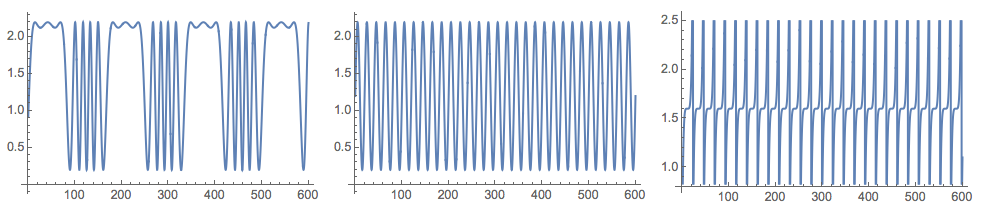``````(*Mixed signals*)
Grid[{Map[ListLinePlot[#, ImageSize -> 250] &, M]}]``````I took the data generation formulas from .

## ICA application

``Import["https://raw.githubusercontent.com/antononcube/MathematicaForPrediction/master/IndependentComponentAnalysis.m"]``

It is important to note that the usual ICA model interpretation for the factorized matrix X is that each column is a variable (audio signal) and each row is an observation (recordings of the microphones at a given time). The matrix 3×1201 M was constructed with the interpretation that each row is a signal, hence we have to transpose M in order to apply the ICA algorithms, X=M^T.

``````X = Transpose[M];

{S, A} = IndependentComponentAnalysis[X, 3];``````

Check the approximation of the obtained factorization:

``````Norm[X - S.A]
(* 3.10715*10^-14 *)``````

Plot the found source signals:

``````Grid[{Map[ListLinePlot[#, PlotRange -> All, ImageSize -> 250] &,
Transpose[S]]}]``````Because of the random initialization of the inverting matrix in the algorithm the result my vary. Here is the plot from another run: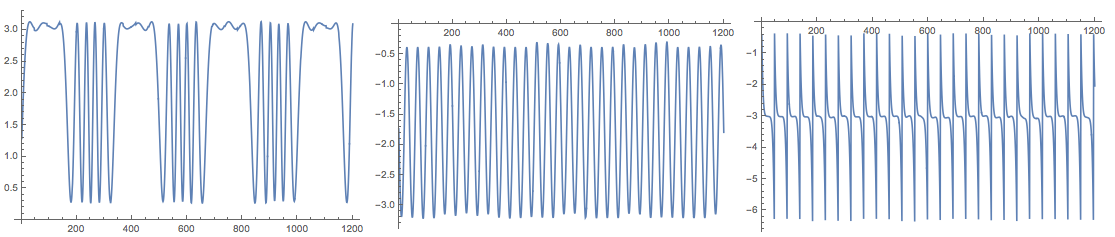The package also provides the function `FastICA` that returns an association with elements that correspond to the result of the function `fastICA` provided by the R package "fastICA". See .

Here is an example usage:

``````res = FastICA[X, 3];

Keys[res]
(* {"X", "K", "W", "A", "S"} *)

Grid[{Map[
ListLinePlot[#, PlotRange -> All, ImageSize -> Medium] &,
Transpose[res["S"]]]}]``````Note that (in adherence to ) the function `FastICA` returns the matrices S and A for the centralized matrix X. This means, for example, that in order to check the approximation proper mean has to be supplied:

``````Norm[X - Map[# + Mean[X] &, res["S"].res["A"]]]
(* 2.56719*10^-14 *)``````

## Signatures and results

The result of the function `IndependentComponentAnalysis` is a list of two matrices. The result of `FastICA` is an association of the matrices obtained by ICA. The function `IndependentComponentAnalysis` takes a method option and options for precision goal and maximum number of steps:

``````In:= Options[IndependentComponentAnalysis]

Out= {Method -> "FastICA", MaxSteps -> 200, PrecisionGoal -> 6}``````

The intent is `IndependentComponentAnalysis` to be the front interface to different ICA algorithms. (Hence, it has a Method option.) The function `FastICA` takes as options the named arguments of the R function `fastICA` described in .

``````In:= Options[FastICA]

Out= {"NonGaussianityFunction" -> Automatic,
"NegEntropyFactor" -> 1, "InitialUnmixingMartix" -> Automatic,
"RowNorm" -> False, MaxSteps -> 200, PrecisionGoal -> 6,
"RFastICAResult" -> True}``````

At this point `FastICA` has only the deflation algorithm described in . ( has also the so called "symmetric" ICA sub-algorithm.) The R function `fastICA` in  can use only two neg-Entropy functions log(cosh(x)) and exp(-u^2/2). Because of the symbolic capabilities of Mathematica `FastICA` of  can take any listable function through the option "NonGaussianityFunction", and it will find and use the corresponding first and second derivatives.

## Using NNMF for ICA

It seems that in some cases, like the generated data used in this blog post, Non-Negative Matrix Factorization (NNMF) can be applied for doing ICA.

To be clear, NNMF does dimension reduction, but its norm minimization process does not enforce variable independence. (It enforces non-negativity.) There are at least several articles discussing modification of NNMF to do ICA. For example .

Load NNMF package  (from MathematicaForPrediction at GitHub):

``Import["https://raw.githubusercontent.com/antononcube/MathematicaForPrediction/master/NonNegativeMatrixFactorization.m"]``

After several applications of NNMF we get signals close to the originals:

``````{W, H} = GDCLS[M, 3];
Grid[{Map[ListLinePlot[#, ImageSize -> 250] &, Normal[H]]}]``````For the generated data in this blog post, FactICA is much faster than NNMF and produces better separation of the signals with every run. The data though is a typical representative for the problems ICA is made for. Another comparison with image de-noising, extending my previous blog post, will be published shortly.

## ICA for mixed time series of city temperatures

Using Mathematica‘s function `WeatherData` we can get temperature time series for a small set of cities over a certain time grid. We can mix those time series into a multi-dimensional signal, MS, apply ICA to MS, and judge the extracted source signals with the original ones.

This is done with the following commands.

### Get time series data

``````cities = {"Sofia", "London", "Copenhagen"};
timeInterval = {{2016, 1, 1}, {2016, 1, 31}};
ts = WeatherData[#, "Temperature", timeInterval] & /@ cities;

opts = {PlotTheme -> "Detailed", FrameLabel -> {None, "temperature,\[Degree]C"}, ImageSize -> 350};
DateListPlot[ts,
PlotLabel -> "City temperatures\nfrom " <> DateString[timeInterval[], {"Year", ".", "Month", ".", "Day"}] <>
" to " <> DateString[timeInterval[], {"Year", ".", "Month", ".", "Day"}],
PlotLegends -> cities, ImageSize -> Large, opts]``````### Cleaning and resampling (if any)

Here we check the data for missing data:

``````Length /@ Through[ts["Path"]]
Count[#, _Missing, \[Infinity]] & /@ Through[ts["Path"]]
Total[%]
(* {1483, 1465, 742} *)
(* {0,0,0} *)
(* 0 *)``````

Resampling per hour:

``ts = TimeSeriesResample[#, "Hour", ResamplingMethod -> {"Interpolation", InterpolationOrder -> 1}] & /@ ts``

### Mixing the time series

In order to do a good mixing we select a mixing matrix for which all column sums are close to one:

``````mixingMat = #/Total[#] & /@ RandomReal[1, {3, 3}];
MatrixForm[mixingMat]
(* mixingMat = {{0.357412, 0.403913, 0.238675}, {0.361481, 0.223506, 0.415013}, {0.36564, 0.278565, 0.355795}} *)
Total[mixingMat]
(* {1.08453, 0.905984, 1.00948} *)``````

Note the row normalization.

Make the mixed signals:

``tsMixed = Table[TimeSeriesThread[mixingMat[[i]].# &, ts], {i, 3}]``

Plot the original and mixed signals:

``````Grid[{{DateListPlot[ts, PlotLegends -> cities, PlotLabel -> "Original signals", opts],
DateListPlot[tsMixed, PlotLegends -> Automatic, PlotLabel -> "Mixed signals", opts]}}]
``````### Application of ICA

At this point we apply ICA (probably more than once, but not too many times) and plot the found source signals:

``````X = Transpose[Through[tsMixed["Path"]][[All, All, 2]] /. Quantity[v_, _] :> v];
{S, A} = IndependentComponentAnalysis[X, 3];
DateListPlot[Transpose[{tsMixed[]["Dates"], #}], PlotTheme -> "Detailed", ImageSize -> 250] & /@ Transpose[S]``````Compare with the original time series:

``MapThread[DateListPlot[#1, PlotTheme -> "Detailed", PlotLabel -> #2, ImageSize -> 250] &, {tsPaths, cities}]``After permuting and inverting some of the found sources signals we see they are fairly good:

``````pinds = {3, 1, 2};
pmat = IdentityMatrix[[All, pinds]];

DateListPlot[Transpose[{tsMixed[]["Dates"], #}], PlotTheme -> "Detailed", ImageSize -> 250] & /@
Transpose[S.DiagonalMatrix[{1, -1, 1}].pmat]``````## References

 A. Hyvarinen and E. Oja (2000) Independent Component Analysis: Algorithms and Applications, Neural Networks, 13(4-5):411-430 . URL: https://www.cs.helsinki.fi/u/ahyvarin/papers/NN00new.pdf .

 Wikipedia entry, Independent component analysis, URL: https://en.wikipedia.org/wiki/Independent_component_analysis .

 A. Antonov, Independent Component Analysis Mathematica package, (2016), source code MathematicaForPrediction at GitHub, package IndependentComponentAnalysis.m .

 J. L. Marchini, C. Heaton and B. D. Ripley, fastICA, R package, URLs: https://cran.r-project.org/web/packages/fastICA/index.html, https://cran.r-project.org/web/packages/fastICA/fastICA.pdf .

 A. Antonov, Implementation of the Non-Negative Matrix Factorization algorithm in Mathematica, (2013), source code at MathematicaForPrediction at GitHub, package NonNegativeMatrixFactorization.m.

 H. Hsieh and J. Chien, A new nonnegative matrix factorization for independent component analysis, (2010), Conference: Acoustics Speech and Signal Processing (ICASSP).

# Comparison of PCA and NNMF over image de-noising

This post compares two dimension reduction techniques Principal Component Analysis (PCA) / Singular Value Decomposition (SVD) and Non-Negative Matrix Factorization (NNMF) over a set of images with two different classes of signals (two digits below) produced by different generators and overlaid with different types of noise.

PCA/SVD produces somewhat good results, but NNMF often provides great results. The factors of NNMF allow interpretation of the basis vectors of the dimension reduction, PCA in general does not. This can be seen in the example below.

## Data

First let us get some images. I am going to use the MNIST dataset for clarity. (And because I experimented with similar data some time ago — see Classification of handwritten digits.).

``````MNISTdigits = ExampleData[{"MachineLearning", "MNIST"}, "TestData"];
{testImages, testImageLabels} =
Transpose[List @@@ RandomSample[Cases[MNISTdigits, HoldPattern[(im_ -> 0 | 4)]], 100]];
testImages
``````See the breakdown of signal classes:

``````Tally[testImageLabels]
(* {{4, 48}, {0, 52}} *)``````

Verify the images have the same sizes:

``````Tally[ImageDimensions /@ testImages]
dims = %[[1, 1]]``````

Add different kinds of noise to the images:

``````noisyTestImages6 =
Table[ImageEffect[
testImages[[i]],
{RandomChoice[{"GaussianNoise", "PoissonNoise", "SaltPepperNoise"}], RandomReal}], {i, Length[testImages]}];
``````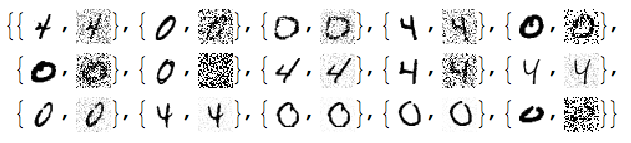Since the important values of the signals are 0 or close to 0 we negate the noisy images:

``````negNoisyTestImages6 = ImageAdjust@*ColorNegate /@ noisyTestImages6
``````## Linear vector space representation

We unfold the images into vectors and stack them into a matrix:

``````noisyTestImagesMat = (Flatten@*ImageData) /@ negNoisyTestImages6;
Dimensions[noisyTestImagesMat]

(* {100, 784} *)
``````

Here is centralized version of the matrix to be used with PCA/SVD:

``````cNoisyTestImagesMat =
Map[# - Mean[noisyTestImagesMat] &, noisyTestImagesMat];
``````

(With NNMF we want to use the non-centralized one.)

Here confirm the values in those matrices:

``````Grid[{Histogram[Flatten[#], 40, PlotRange -> All,
ImageSize -> Medium] & /@ {noisyTestImagesMat,
cNoisyTestImagesMat}}]
``````## SVD dimension reduction

For more details see the previous answers.

``````{U, S, V} = SingularValueDecomposition[cNoisyTestImagesMat, 100];
ListPlot[Diagonal[S], PlotRange -> All, PlotTheme -> "Detailed"]
dS = S;
Do[dS[[i, i]] = 0, {i, Range[10, Length[S], 1]}]
newMat = U.dS.Transpose[V];
denoisedImages =
Map[Image[Partition[# + Mean[noisyTestImagesMat], dims[]]] &, newMat];
``````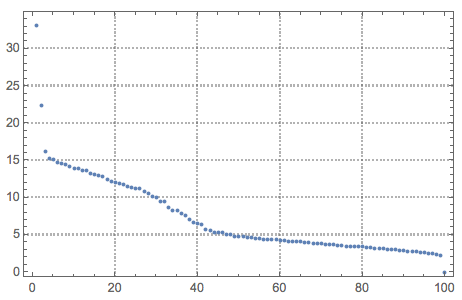Here are how the new basis vectors look like:

``````Take[#, 50] &@
Dimensions[V][]], Diagonal[S], Transpose[V]}]
``````Basically, we cannot tell much from these SVD basis vectors images.

Here we load the packages for what is computed next:

``````Import["https://raw.githubusercontent.com/antononcube/MathematicaForPrediction/master/NonNegativeMatrixFactorization.m"]

Import["https://raw.githubusercontent.com/antononcube/MathematicaForPrediction/master/OutlierIdentifiers.m"]
``````

The blog post “Statistical thesaurus from NPR podcasts” discusses an example application of NNMF and has links to documents explaining the theory behind the NNMF utilization.

The outlier detection package is described in a previous blog post “Outlier detection in a list of numbers”.

## NNMF

This command factorizes the image matrix into the product W H :

``````{W, H} = GDCLS[noisyTestImagesMat, 20, "MaxSteps" -> 200];
{W, H} = LeftNormalizeMatrixProduct[W, H];

Dimensions[W]
Dimensions[H]

(* {100, 20} *)
(* {20, 784} *)
``````

The rows of H are interpreted as new basis vectors and the rows of W are the coordinates of the images in that new basis. Some appropriate normalization was also done for that interpretation. Note that we are using the non-normalized image matrix.

Let us see the norms of \$H\$ and mark the top outliers:

``````norms = Norm /@ H;
ListPlot[norms, PlotRange -> All, PlotLabel -> "Norms of H rows",
PlotTheme -> "Detailed"] //
ColorPlotOutliers[TopOutliers@*HampelIdentifierParameters]
OutlierPosition[norms, TopOutliers@*HampelIdentifierParameters]
OutlierPosition[norms, TopOutliers@*SPLUSQuartileIdentifierParameters]
``````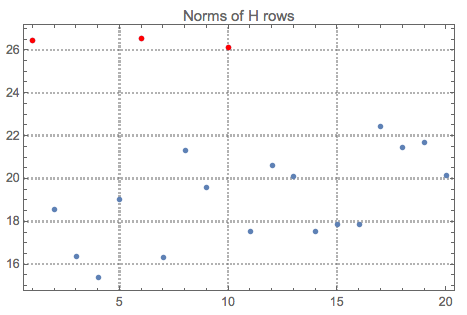Here is the interpretation of the new basis vectors (the outliers are marked in red):

``````MapIndexed[{#2[], Norm[#], Image[Partition[#, dims[]]]} &, H] /. (# -> Style[#, Red] & /@
OutlierPosition[norms, TopOutliers@*HampelIdentifierParameters])
``````Using only the outliers of \$H\$ let us reconstruct the image matrix and the de-noised images:

``````pos = {1, 6, 10}
dHN = Total[norms]/Total[norms[[pos]]]*
DiagonalMatrix[
ReplacePart[ConstantArray[0, Length[norms]],
Map[List, pos] -> 1]];
newMatNNMF = W.dHN.H;
denoisedImagesNNMF =
Map[Image[Partition[#, dims[]]] &, newMatNNMF];
``````

Often we cannot just rely on outlier detection and have to hand pick the basis for reconstruction. (Especially when we have more than one classes of signals.)

## Comparison

At this point we can plot all images together for comparison:

``````imgRows =
Transpose[{testImages, noisyTestImages6,
With[{ncol = 5},
Grid[Prepend[Partition[imgRows, ncol],
Style[#, Blue, FontFamily -> "Times"] & /@
Table[{"original", "noised", "SVD", "NNMF"}, ncol]]]]
``````We can see that NNMF produces cleaner images. This can be also observed/confirmed using threshold binarization — the NNMF images are much cleaner.

``````imgRows =
With[{th = 0.5},
Binarize[#4, th]} &, {testImageLabels, noisyTestImages6,
With[{ncol = 5},
Grid[Prepend[Partition[imgRows, ncol],
Style[#, Blue, FontFamily -> "Times"] & /@
Table[{"label", "noised", "SVD", "NNMF"}, ncol]]]]
``````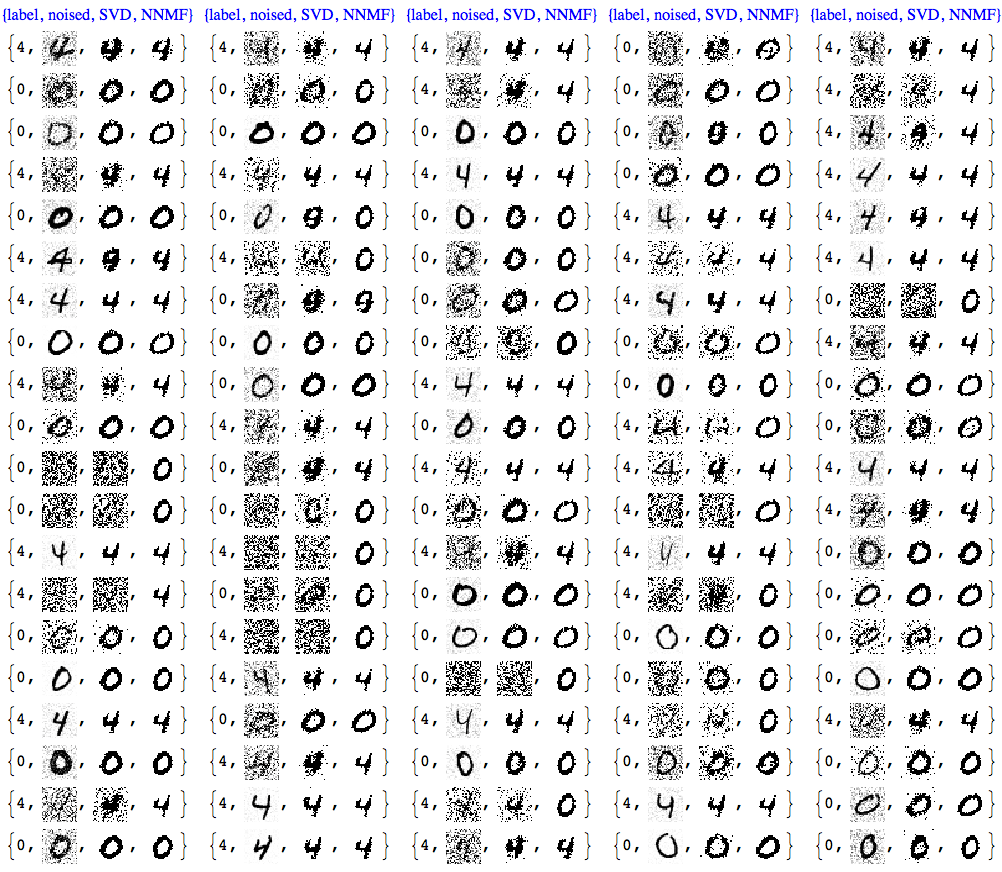Usually with NNMF in order to get good results we have to do more that one iteration of the whole factorization and reconstruction process. And of course NNMF is much slower. Nevertheless, we can see clear advantages of NNMF’s interpretability and leveraging it.

## Gallery with other experiments

In those experiments I had to hand pick the NNMF basis used for the reconstruction. Using outlier detection without supervision would not produce good results most of the time.

## Further comparison with Classify

We can further compare the de-noising results by building signal (digit) classifiers and running them over the de-noised images.

For such a classifier we have to decide:

1. do we train only with images of the two signal classes or over a larger set of signals;
2. how many signals we train with;
3. with what method the classifiers are built.

Below I use the default method of `Classify` with all digit images in MNIST that are not in the noised images set. The classifier is run with the de-noising traced these 0-4 images:

Get the images:

``````{trainImages, trainImageLabels} = Transpose[List @@@ Cases[MNISTdigits, HoldPattern[(im_ -> _)]]];
pred = Map[! MemberQ[testImages, #] &, trainImages];
trainImages = Pick[trainImages, pred];
trainImageLabels = Pick[trainImageLabels, pred];
Tally[trainImageLabels]

(* {{0, 934}, {1, 1135}, {2, 1032}, {3, 1010}, {4, 928}, {5, 892}, {6, 958}, {7, 1028}, {8, 974}, {9, 1009}} *)``````
``` ```

Build the classifier:

``````digitCF = Classify[trainImages -> trainImageLabels]
``````
``` ```

Compute classifier measurements for the original, the PCA de-noised, and NNMF de-noised images:

``````origCM = ClassifierMeasurements[digitCF, Thread[(testImages) -> testImageLabels]]

pcaCM = ClassifierMeasurements[digitCF,

nnmfCM = ClassifierMeasurements[digitCF,
``` ```
``````Grid[{{"Original", "PCA", "NNMF"},
``` ```